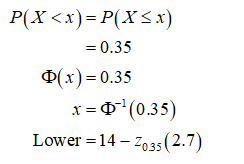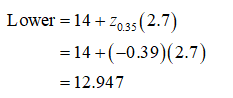# On average, indoor cats live to 14 years old with a standard deviation of 2.7 years. Suppose that the distribution is normal. Let X = the age at death of a randomly selected indoor cat. Round answers to 4 decimal places where possible. c.  The middle 30% of indoor cats' age of death lies between what two numbers?     Low: years      High: years

Question
29 views

On average, indoor cats live to 14 years old with a standard deviation of 2.7 years. Suppose that the distribution is normal. Let X = the age at death of a randomly selected indoor cat. Round answers to 4 decimal places where possible.

c.  The middle 30% of indoor cats' age of death lies between what two numbers?
Low: years
High: years

check_circle

Step 1

Here, X follows the normal distribution with mean 14 years old and a standard deviation of 2.7 years. Let X be the age at death of a randomly selected indoor cat.

Compute the middle 30% of indoor cats’ age of death lies between the two numbers:

First finding the z-score for the 35 percentiles.

Therefore,Using the standard normal table, choose the corresponding row and column value nearer to the probability of 0.35. the z-score corresponding value nearer to 0.35 is -0.39....

### Want to see the full answer?

See Solution

#### Want to see this answer and more?

Solutions are written by subject experts who are available 24/7. Questions are typically answered within 1 hour.*

See Solution
*Response times may vary by subject and question.
Tagged in